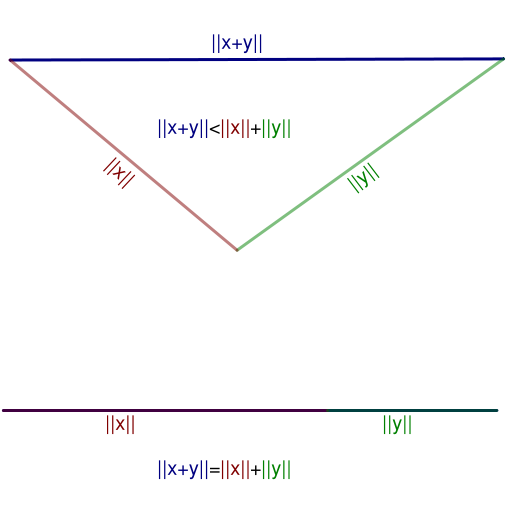# A Triangle InequalityLet a triangle with sides of length $a,b,c$ have perimeter $2$. What is the maximum value of $k$ such that $\dfrac{1-a}{b}+\dfrac{1-b}{c}+\dfrac{1-c}{a}\ge k$ is always true? Prove your claim.

×